Next: Bibliography Up: CVonline_ANOVA Previous: CVonline_ANOVA

# One way ANOVA - Analysis of variance

Analysis of variance (ANalysis Of VAriance) is a general method for studying sampled-data relationships [1,2]. The method enables the difference between two or more sample means to be analysed, achieved by subdividing the total sum of squares. One way ANOVA is the simplest case. The purpose is to test for significant differences between class means, and this is done by analysing the variances. Incidentally, if we are only comparing two different means then the method is the same as the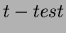for independent samples. The basis of ANOVA is the partitioning of sums of squares into between-class (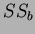) and within-class (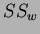). It enables all classes to be compared with each other simultaneously rather than individually; it assumes that the samples are normally distributed. The one way analysis is calculated in three steps, first the sum of squares for all samples, then the within class and between class cases. For each stage the degrees of freedom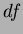are also determined, whereis the number of independent pieces of information' that go into the estimate of a parameter. These calculations are used via the Fisher statistic to analyse the null hypothesis. The null hypothesis states that there are no differences between means of different classes, suggesting that the variance of the within-class samples should be identical to that of the between-class samples (resulting in no between-class discrimination capability). It must however be noted that small sample sets will produce random fluctuations due to the assumption of a normal distribution. If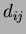is the sample for the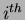class and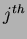data point then the total sum of squares is defined as: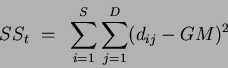(1)

with degrees of freedom: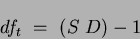(2)

where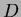is the number of data points (assuming equal numbers of data points in each class) and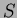is the number of classes and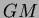is the grand mean: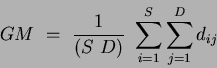(3)

The second stage determines the sum of squares for the within class case, defined as: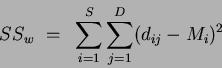(4)

where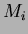is theclass mean determined by: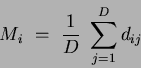(5)

and the within classis: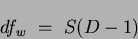(6)

The sum of squares for the between class case is: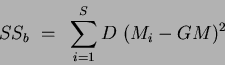(7)

with the correspondingof: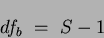(8)

Defining the total degrees of freedom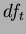and the total sum of squares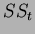as: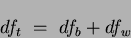(9)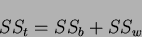(10)

Finally if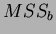is the mean square deviations (or variances) for the between class case, and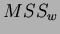is the reciprocal for the within class case then: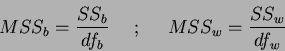(11)

It is now possible to evaluate the null hypothesis using the Fisher or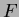Fisher statistic statistic, defined as: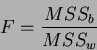(12)

If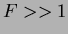then it is likely that differences between class means exist. These results are then tested for statistical significance or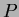-value, where the-value is the probability that a variate would assume a value greater than or equal to the value observed strictly by chance. If the-value is small (eg.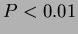or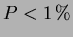) then this implies that the means differ by more than would be expected by chance alone. By setting a limit on the-value, (i.e.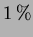) a criticalvalue can be determined. The critical value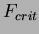is determined (via standard lookup tables) through the between-class (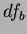) and within-class (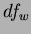)values. Values ofgreater than the critical value denote the rejection of the null hypothesis, which prompts further investigation into the nature of the differences of the class means. In this way ANOVA can be used to prune a list of features. Figure 0.1 shows exampleFisher statistic critical value values for a lowdistribution (i.e. a small dataset). Asincreases (i.e. the dataset size increases) thedistribution will become tighter' and more peaked in appearance, while the peak will shift away from the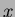axis towards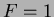.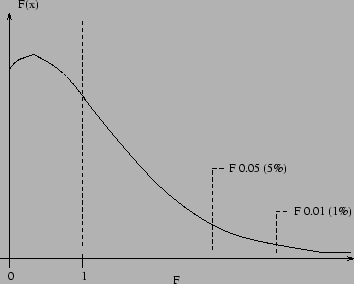Thevalue gives a reliable test for the null hypothesis, but it cannot indicate which of the means is responsible for a significantly low probability. To investigate the cause of rejection of the null hypothesis post-hoc or multiple comparison tests can be used. These examine or compare more than one pair of means simultaneously. Here we use the Scheffe post-hoc test. This tests all pairs for differences between means and all possible combinations of means. The test statistic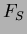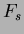Scheffe post-hoc test value is: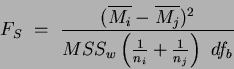(13)

where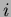and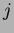are the classes being compared and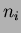andare the number of samples and mean of class, respectively. If the number of samples (data points) is the same for all classes then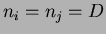. The test statistic is calculated for each pair of means and the null hypothesis is again rejected ifis greater than the critical value, as previously defined for the original ANOVA analysis. This Scheffe post hoc test is known to be conservative which helps to compensate for spurious significant results that occur with multiple comparisons . The test gives a measure of the difference between all means for all combinations of means.

In terms of classification, largestatistic values do not necessarily indicate useful features. They only indicate a well spread feature space, which for a large dataset is a positive attribute. It suggests that the feature has scope or room' for more classes to be added to the dataset. Equally, features with smallervalues (but greater than the critical values) may separate a portion of the dataset, which was previously confused' with another portion. Adding this new feature, increasing the feature space dimensions, may prove beneficial. In this manner, features which appear `less good' (i.e. lowerstatistic values than alternative features) may, in fact, prove useful in terms of classification.Next: Bibliography Up: CVonline_ANOVA Previous: CVonline_ANOVA
Jamie Shutler 2002-08-15